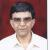true

Learn Python Training from the Best Tutors

•Affordable fees
•1-1 or Group class
•Flexible Timings
•Verified Tutors

Search in

Writing Simple Programs in PythonAshish K Sharma
26/11/2021 00

Writing a program, especially for a beginner, is a challenging and daunting task! He/She knows the language but is not able to start. To make things simple, it is advisable to follow a process that is a correct program. Let us take a look at the Software Development Process,a systematic approach to writing a program.

SOFTWARE DEVELOPMENT PROCESS.

1. Formulate requirements

We have to understand the problem first, thoroughly, in order to program. Unless we dont understand the problem, how can we try to solve it?

2. Determine Specifications.

One has to describe exactly what our program is supposed to do. What are its inputs/outputs? We will not bother, at this stage, about how the program runs, but instead, what it will accomplish.

3. Create a Design

Here, the actual program is designed. The algorithm which will meet our specifications is designed here.

4. Implement the design

Now, we will translate our design into an actual Python(or any other language) program.

5. Testing/Debugging

This is an important step. Very rarely will your program run the first time correctly round or produce the results you expected. There are bound to be errors and at this stage,the program is carefully debugged,its underlying algorithm reviewed.

6. Maintenance.

The needs of the user are ever-evolving. So should your program! Many of the programs are rarely finished. They are constantly being updated.

Now, let us take an example to illustrate the process. Suppose we want to calculate the future value of an investment. Or, how much will an amount be 10 years from now? It depends on the initial amount and the interest rate. Given the principal and the interest rate, a program should be able to calculate the future value of an investment. Thus, we have defined the problem. Now, let us develop the specifications of the program.

As far as the inputs are concerned, we require the following:

1. The amount of money being invested(in rupees) ---- The Principal

2. The annual interest rate, expressed as a percentage--- APR

and the output of the program will be:

3. The value of the investment is 10 years in the future.

Our algorithm, which we will design now, depends upon the following formula:

Value after 1 year: Principal(1+APR), applied 10 times.

Algorithms are written in Pseudo Code. The following is the algorithm:

Print an introduction

Input the amount of the principal (principal)

Input the annual percentage rate (apr)

Repeat 10 times:

principal = principal * (1 + apr)

Output the value of principal

Note: The future value can be calculated in one step also. Here, the loop is being used just to illustrate the concept of programming in detail for beginners. If we know how to calculate the interest for one year, we can calculate the interest any number of years into the future.

Now, let us write the actual program in Python. Here is the complete program:

# futval . py

# A program to compute the value of an investment

# carried 10 years into the future

print ("This program calculates the future value")

print ("of a 10-year investment.")

principal = input ("Enter the initial principal: ")

apr = input ("Enter the annual interest rate: ")

for i in range (10) :

principal = principal * (1 + apr)

print ("The value in 10 years is: ", principal)

The actual running of the program:

This program calculates the future value

of a 10-year investment.

Enter the initial principal: 5000

Enter the annual interest rate: 0.08

('The value in 10 years is: ', 10794.624986363942)

Note: This lesson essentially focuses on an orderly software development process. To write a program in any language, we had to learn the elements of the language, its basic building blocks, syntax and semantics.

0 Dislike
Follow 2Other Lessons for You

File Handling in Python - Basic Concept
File (Flat) Handling in Python Types of files in python: 1: Text file: Stores data in the form of characters. Customarily used to store text/string data. 2: Binary file: Stores data in the form of bytes....DBMS - SQL - Any/All
All - Operator SELECT empno, sal FROM emp WHERE sal > ALL (1999, 2999, 3999); Output of Above query is same as below query SELECT empno, sal FROM emp WHERE sal > 1999 AND sal > 2999...Write your first Python program in 10 minutesCode: Gantt Chart: Horizontal bar using matplotlib for tasks with Start Time and End Time
import pandas as pd from datetime import datetimeimport matplotlib.dates as datesimport matplotlib.pyplot as plt def gantt_chart(df_phase): # Now convert them to matplotlib's internal format... ...Looking for Python Training classes?

Learn from Best Tutors on UrbanPro.

Are you a Tutor or Training Institute?

Join UrbanPro Today to find students near you
X

Looking for Python Training Classes?

The best tutors for Python Training Classes are on UrbanPro

• Select the best Tutor
• Book & Attend a Free Demo
• Pay and start LearningLearn Python Training with the Best Tutors

The best Tutors for Python Training Classes are on UrbanProUrbanPro.com is India's largest network of most trusted tutors and institutes. Over 55 lakh students rely on UrbanPro.com, to fulfill their learning requirements across 1,000+ categories. Using UrbanPro.com, parents, and students can compare multiple Tutors and Institutes and choose the one that best suits their requirements. More than 7.5 lakh verified Tutors and Institutes are helping millions of students every day and growing their tutoring business on UrbanPro.com. Whether you are looking for a tutor to learn mathematics, a German language trainer to brush up your German language skills or an institute to upgrade your IT skills, we have got the best selection of Tutors and Training Institutes for you. Read more